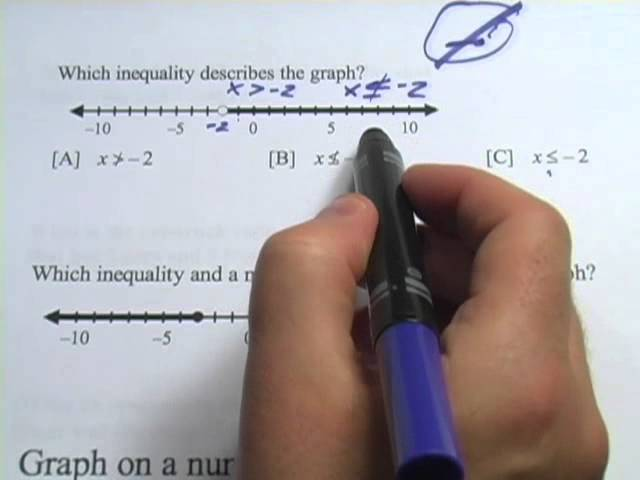# Inequalities: A Guide to NegationTLDR When negating an inequality, the sign is flipped and the equal sign is removed.

## Key insights

• 🚫
Negated inequalities are like "no smoking" signs, indicating that x is not greater than a certain value.
• 🔄
Turning an inequality around can result in a negated inequality, as demonstrated in the example.
• 🔄
Negating an inequality can change the direction of the inequality, as seen in the example where "x is not greater than or equal to negative 4" becomes "x is less than negative four."

## Q&A

• What is the key idea of the video?

The key idea of the video is that when negating an inequality, the sign is flipped and the equal sign is removed.

• How should the sign be changed when negating an inequality?

When negating an inequality, the sign should be flipped.

• What happens to the equal sign when negating an inequality?

When negating an inequality, the equal sign is removed.

• Is it necessary to change the sign when negating an inequality?

Yes, it is necessary to change the sign when negating an inequality.

• What is the significance of removing the equal sign when negating an inequality?

Removing the equal sign when negating an inequality helps to accurately represent the relationship between the two quantities being compared.

## Timestamped Summary

• 📉
00:00
x is not greater than negative two, similar to a no smoking sign with a slash through it.
• 📝
00:25
The graph represents the inequality x > -2, but the negated inequality is x ≤ -2.
• 📝
00:47
The negated inequality for the given graph is x is not less than or equal to negative 2.
• 📝
01:24
The negated inequality is "x is not greater than negative 3."
• 📝
02:04
Flipping the sign and removing the equal sign can be a helpful shortcut when dealing with negated inequalities.Report the articleThank you for the feedback